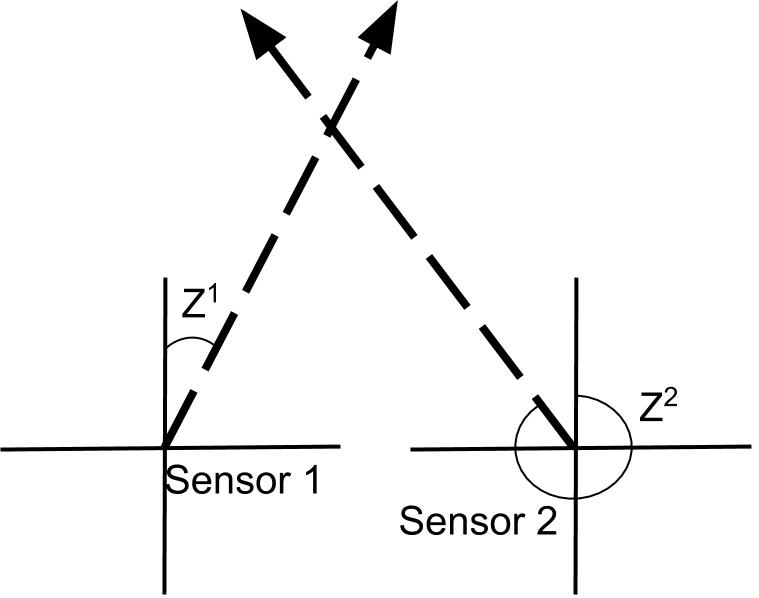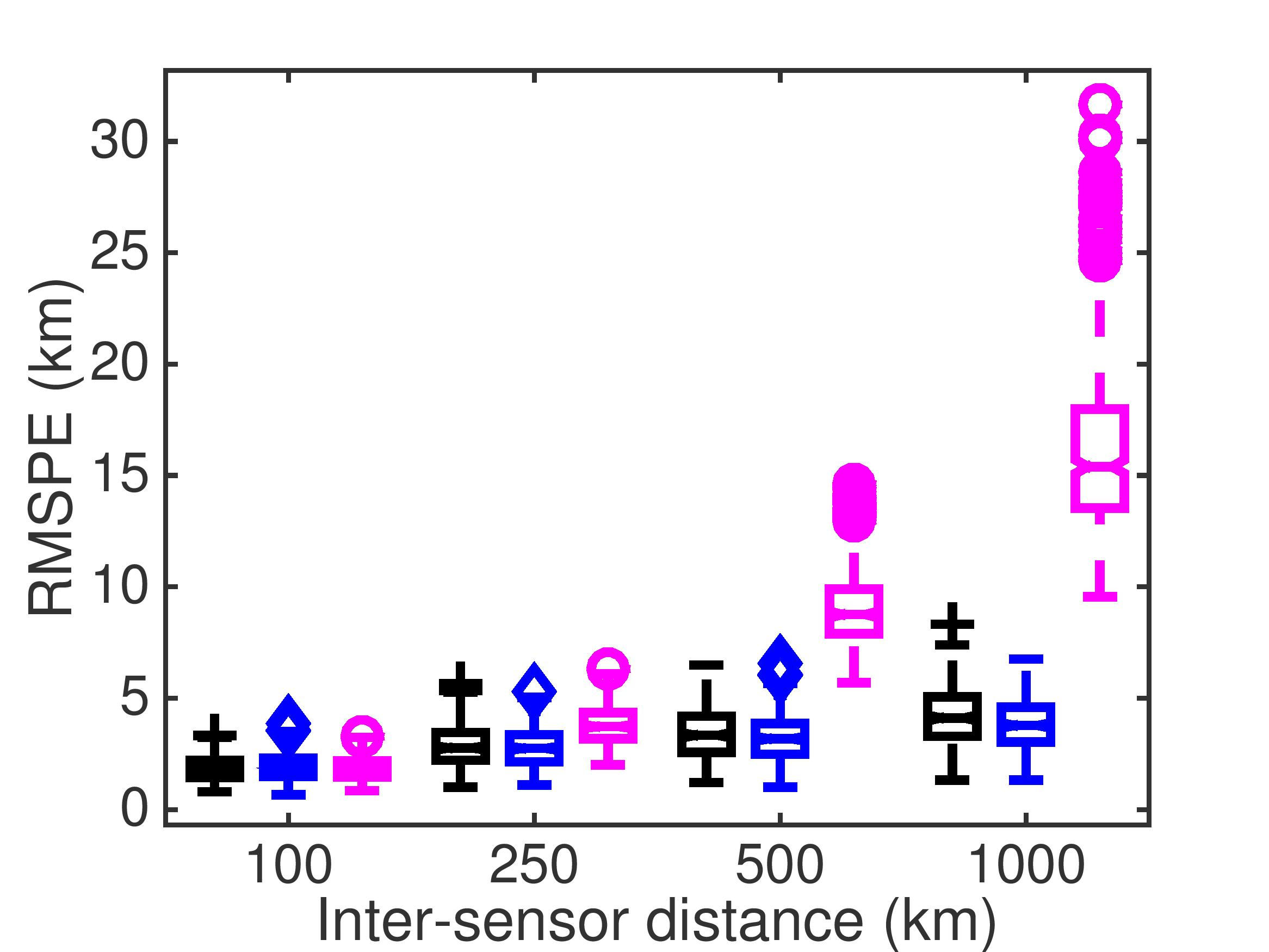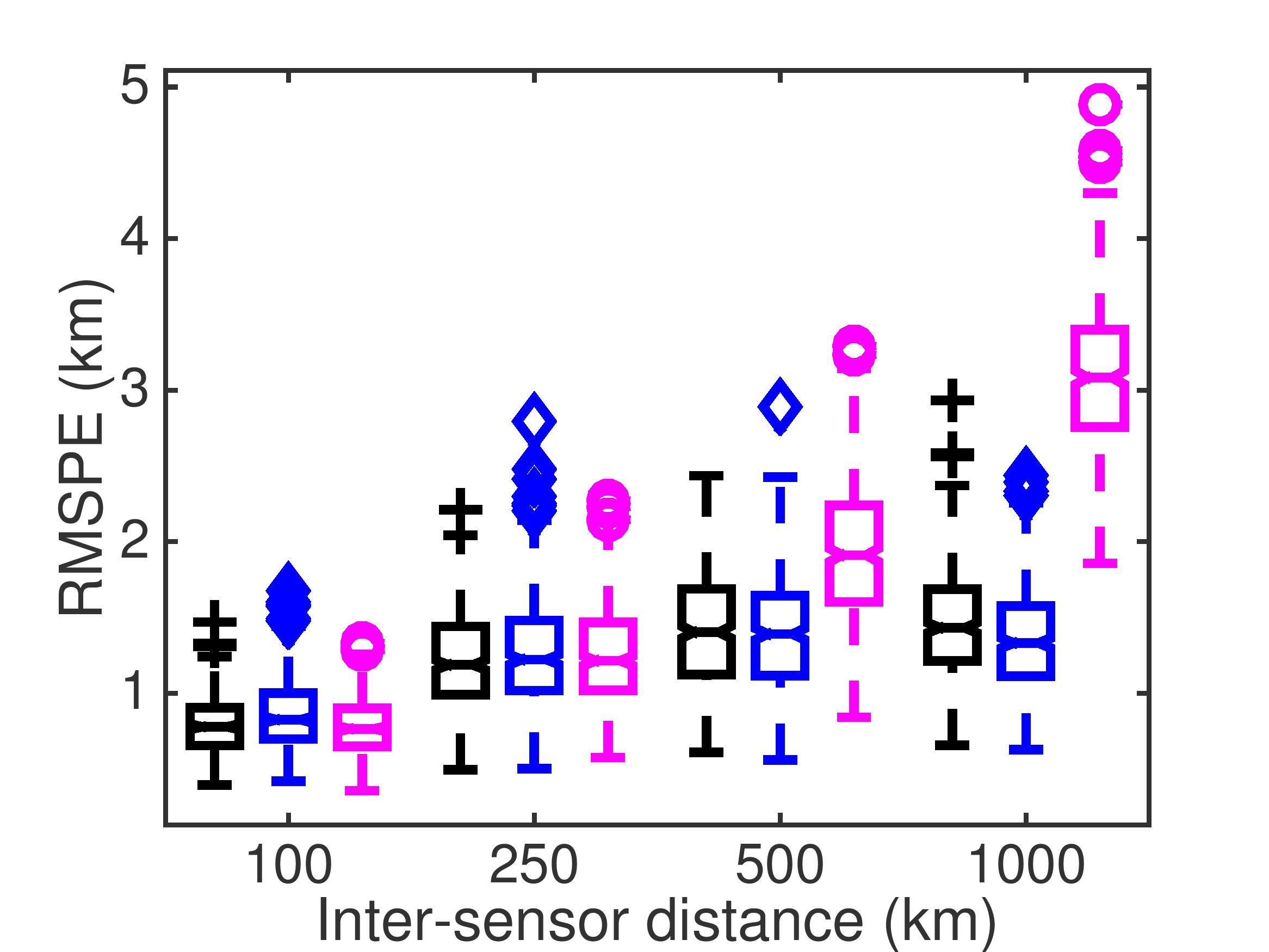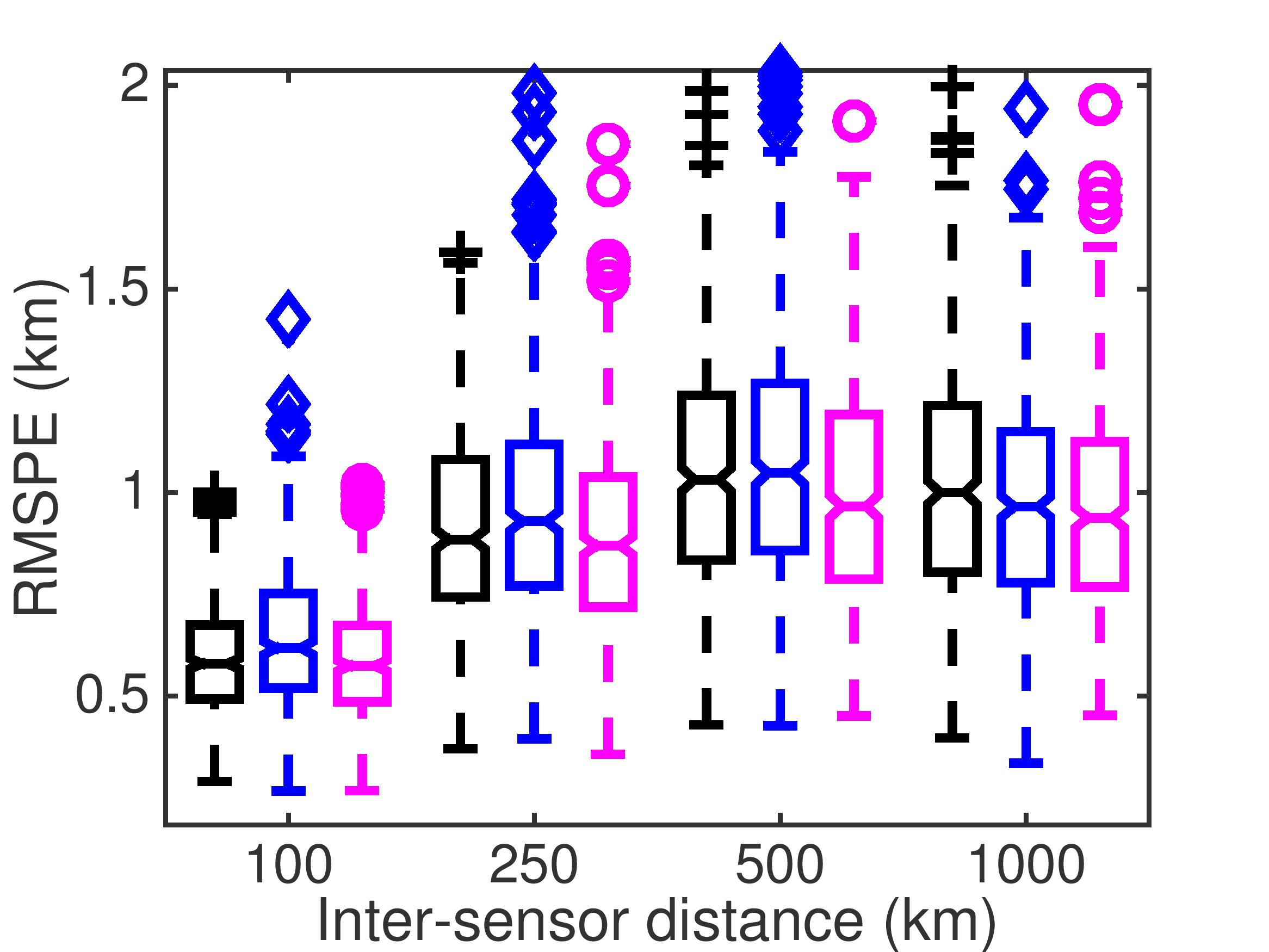## Bearing-only Single Target Tracking on Spherical Surfaces

Bearing-only tracking is a key task in many applications such as submarine tracking, aircraft surveillance, and whale migration monitoring, etc.  The objective is to track a maneuvering target using only noisy bearing (or degree-of-arrival) measurements.  Fig. 1 illustrates the concept using two sensors with bearings Z1 and Z2 respectively.

A standard assumption in current literature is that both sensors and targets are placed on a flat plane and the bearing angles are calculated accordingly. However, because the Earth is not flat, this assumption quickly breaks down in real world applications and leads to significant error in bearing calculations. In this project, we aim to develop a distributed tracking algorithm that takes into consideration Earth's curvature in order to provide improved performance with minimal communication overhead.Fig. 1 Illustration of bearing-only tracking

Given the bearing measurement model on spherical surface, we compute an approximate likelihood function that can be conveniently factorized.

$\log p(z^1,...z^K|x^i) \approx -\sum_{j=1}^6 \left(G_j(x^i)\sum_{k=1}^K\frac{C_j^k}{2\hat{R}_k} \right)$

The terms $\frac{C_j^k}{2\hat{R}_k}$ depend only on the coordinates of sensor k and bearing $z^k$, and the terms $G_j(x^i)$ depend only on the particle $x^i$. Thus, sensors only need to compute the six inner sums  using gossip or other distributed methods to obtain the likelihood function.

Our method's overhead depends only on the number of sensors. In comparison, basic particle filters (such as bootstrap) requires reaching consensus on all invidual particle weights across all sensors.

We generate target tracks in three different geographical locations and evaluate algorithm's performance with respect to sensor-target distance. The proposed algorithm (magenta) is on-par with centralized baseline (black); whereas the planar bearing model (blue) breaks down over long distance.Greenland                                                            North Atlantic Ocean                                                  South Atlantic Ocean

Faculty: Prof. Michael Rabbat, Prof. Mark Coates

PhD. Student: Jun Ye Yu

Collaborator: Stephane Blouin, DRDC Atlantic

IEEE Signal Processing Letters, March 2016.

Canadian Conference on Electrical and Computer Engineering, May 2016.